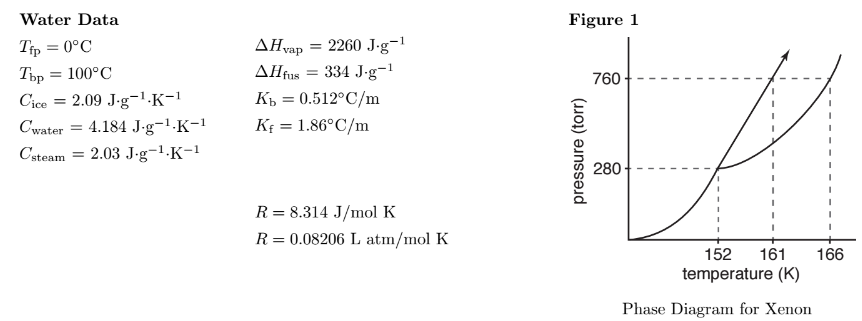# Problem: According to Figure 1, what is the normal boiling point of Xe?A. &lt; 152 KB. 161 KC. 152 KD. 166 KE. &gt; 166 K

###### FREE Expert Solution
95% (271 ratings)
###### Problem Details

According to Figure 1, what is the normal boiling point of Xe?

A. < 152 K

B. 161 K

C. 152 K

D. 166 K

E. > 166 KWhat scientific concept do you need to know in order to solve this problem?

Our tutors have indicated that to solve this problem you will need to apply the Phase Diagram concept. You can view video lessons to learn Phase Diagram. Or if you need more Phase Diagram practice, you can also practice Phase Diagram practice problems.

What is the difficulty of this problem?

Our tutors rated the difficulty ofAccording to Figure 1, what is the normal boiling point of X...as low difficulty.

How long does this problem take to solve?

Our expert Chemistry tutor, Jules took 1 minute and 37 seconds to solve this problem. You can follow their steps in the video explanation above.

What professor is this problem relevant for?

Based on our data, we think this problem is relevant for Professor Bryant's class at USF.